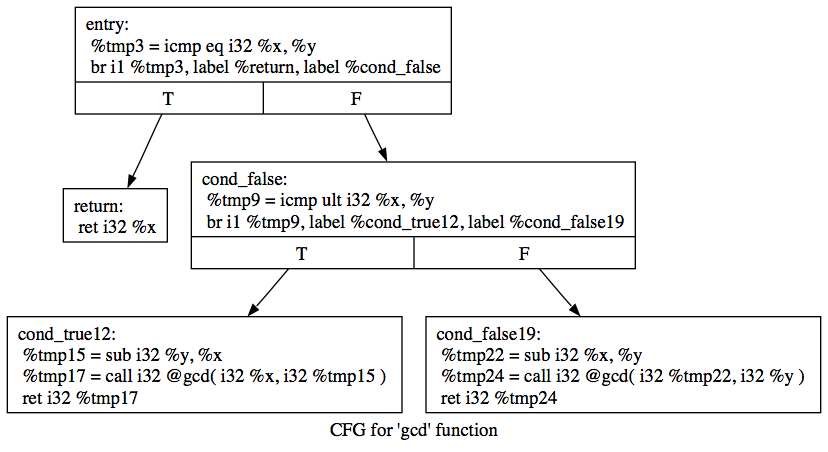LLVM Tutorial 2: A More Complicated Function

Written by Owen Anderson

A First Function

Now that we understand the basics of creating functions in LLVM, let's move on to a more complicated example: something with control flow. As an example, let's consider Euclid's Greatest Common Denominator (GCD) algorithm:

```unsigned gcd(unsigned x, unsigned y) {
if(x == y) {
return x;
} else if(x < y) {
return gcd(x, y - x);
} else {
return gcd(x - y, y);
}
}
```

With this example, we'll learn how to create functions with multiple blocks and control flow, and how to make function calls within your LLVM code. For starters, consider the diagram below.This is a graphical representation of a program in LLVM IR. It places each basic block on a node of a graph and uses directed edges to indicate flow control. These blocks will be serialized when written to a text or bitcode file, but it is often useful conceptually to think of them as a graph. Again, if you are unsure about the code in the diagram, you should skim through the LLVM Language Reference Manual and convince yourself that it is, in fact, the GCD algorithm.

The first part of our code is practically the same as from the first tutorial. The same basic setup is required: creating a module, verifying it, and running the `PrintModulePass` on it. Even the first segment of `makeLLVMModule()` looks essentially the same, except that `gcd` takes one fewer parameter than `mul_add`.

```#include "llvm/Module.h"
#include "llvm/Function.h"
#include "llvm/PassManager.h"
#include "llvm/Analysis/Verifier.h"
#include "llvm/Assembly/PrintModulePass.h"
#include "llvm/Support/IRBuilder.h"
#include "llvm/Support/raw_ostream.h"

using namespace llvm;

Module* makeLLVMModule();

int main(int argc, char**argv) {
Module* Mod = makeLLVMModule();

verifyModule(*Mod, PrintMessageAction);

PassManager PM;
PM.run(*Mod);

delete Mod;
return 0;
}

Module* makeLLVMModule() {
Module* mod = new Module("tut2");

Constant* c = mod->getOrInsertFunction("gcd",
IntegerType::get(32),
IntegerType::get(32),
IntegerType::get(32),
NULL);
Function* gcd = cast<Function>(c);

Function::arg_iterator args = gcd->arg_begin();
Value* x = args++;
x->setName("x");
Value* y = args++;
y->setName("y");
```

Here, however, is where our code begins to diverge from the first tutorial. Because `gcd` has control flow, it is composed of multiple blocks interconnected by branching (`br`) instructions. For those familiar with assembly language, a block is similar to a labeled set of instructions. For those not familiar with assembly language, a block is basically a set of instructions that can be branched to and is executed linearly until the block is terminated by one of a small number of control flow instructions, such as `br` or `ret`.

Blocks correspond to the nodes in the diagram we looked at in the beginning of this tutorial. From the diagram, we can see that this function contains five blocks, so we'll go ahead and create them. Note that we're making use of LLVM's automatic name uniquing in this code sample, since we're giving two blocks the same name.

```  BasicBlock* entry = BasicBlock::Create(getGlobalContext(), ("entry", gcd);
BasicBlock* ret = BasicBlock::Create(getGlobalContext(), ("return", gcd);
BasicBlock* cond_false = BasicBlock::Create(getGlobalContext(), ("cond_false", gcd);
BasicBlock* cond_true = BasicBlock::Create(getGlobalContext(), ("cond_true", gcd);
BasicBlock* cond_false_2 = BasicBlock::Create(getGlobalContext(), ("cond_false", gcd);
```

Now we're ready to begin generating code! We'll start with the `entry` block. This block corresponds to the top-level if-statement in the original C code, so we need to compare `x` and `y`. To achieve this, we perform an explicit comparison using `ICmpEQ`. `ICmpEQ` stands for an integer comparison for equality and returns a 1-bit integer result. This 1-bit result is then used as the input to a conditional branch, with `ret` as the `true` and `cond_false` as the `false` case.

```  IRBuilder<> builder(entry);
Value* xEqualsY = builder.CreateICmpEQ(x, y, "tmp");
builder.CreateCondBr(xEqualsY, ret, cond_false);
```

Our next block, `ret`, is pretty simple: it just returns the value of `x`. Recall that this block is only reached if `x == y`, so this is the correct behavior. Notice that instead of creating a new `IRBuilder` for each block, we can use `SetInsertPoint` to retarget our existing one. This saves on construction and memory allocation costs.

```  builder.SetInsertPoint(ret);
builder.CreateRet(x);
```

`cond_false` is a more interesting block: we now know that ```x != y```, so we must branch again to determine which of `x` and `y` is larger. This is achieved using the `ICmpULT` instruction, which stands for integer comparison for unsigned less-than. In LLVM, integer types do not carry sign; a 32-bit integer pseudo-register can be interpreted as signed or unsigned without casting. Whether a signed or unsigned interpretation is desired is specified in the instruction. This is why several instructions in the LLVM IR, such as integer less-than, include a specifier for signed or unsigned.

Also note that we're again making use of LLVM's automatic name uniquing, this time at a register level. We've deliberately chosen to name every instruction "tmp" to illustrate that LLVM will give them all unique names without getting confused.

```  builder.SetInsertPoint(cond_false);
Value* xLessThanY = builder.CreateICmpULT(x, y, "tmp");
builder.CreateCondBr(xLessThanY, cond_true, cond_false_2);
```

Our last two blocks are quite similar; they're both recursive calls to `gcd` with different parameters. To create a call instruction, we have to create a `vector` (or any other container with `InputInterator`s) to hold the arguments. We then pass in the beginning and ending iterators for this vector.

```  builder.SetInsertPoint(cond_true);
Value* yMinusX = builder.CreateSub(y, x, "tmp");
std::vector<Value*> args1;
args1.push_back(x);
args1.push_back(yMinusX);
Value* recur_1 = builder.CreateCall(gcd, args1.begin(), args1.end(), "tmp");
builder.CreateRet(recur_1);

builder.SetInsertPoint(cond_false_2);
Value* xMinusY = builder.CreateSub(x, y, "tmp");
std::vector<Value*> args2;
args2.push_back(xMinusY);
args2.push_back(y);
Value* recur_2 = builder.CreateCall(gcd, args2.begin(), args2.end(), "tmp");
builder.CreateRet(recur_2);

return mod;
}
```

And that's it! You can compile and execute your code in the same way as before, by doing:

```# c++ -g tut2.cpp `llvm-config --cxxflags --ldflags --libs core` -o tut2
# ./tut2
```Owen Anderson
The LLVM Compiler Infrastructure